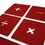Prove: 2=1

Let a=1 and b=1. Now, we have

a=b

or, $a^{2}$ = ab

or, $a^{2}$ - $b^{2}$= ab-$b^{2}$

or, (a+b)(a-b) = b(a-b)

or, (a+b)=b

Thus on putting the values we get,

1+1=1

or, 2=1Note by Bodhisatwa Nandi
6 years, 5 months ago

This discussion board is a place to discuss our Daily Challenges and the math and science related to those challenges. Explanations are more than just a solution — they should explain the steps and thinking strategies that you used to obtain the solution. Comments should further the discussion of math and science.

When posting on Brilliant:

• Use the emojis to react to an explanation, whether you're congratulating a job well done , or just really confused .
• Ask specific questions about the challenge or the steps in somebody's explanation. Well-posed questions can add a lot to the discussion, but posting "I don't understand!" doesn't help anyone.
• Try to contribute something new to the discussion, whether it is an extension, generalization or other idea related to the challenge.
• Stay on topic — we're all here to learn more about math and science, not to hear about your favorite get-rich-quick scheme or current world events.

MarkdownAppears as
*italics* or _italics_ italics
**bold** or __bold__ bold

- bulleted
- list

• bulleted
• list

1. numbered
2. list

1. numbered
2. list
Note: you must add a full line of space before and after lists for them to show up correctly
paragraph 1

paragraph 2

paragraph 1

paragraph 2

> This is a quote
This is a quote
# I indented these lines
# 4 spaces, and now they show
# up as a code block.

print "hello world"
# I indented these lines
# 4 spaces, and now they show
# up as a code block.

print "hello world"
MathAppears as
Remember to wrap math in $$...$$ or $...$ to ensure proper formatting.
2 \times 3 $2 \times 3$
2^{34} $2^{34}$
a_{i-1} $a_{i-1}$
\frac{2}{3} $\frac{2}{3}$
\sqrt{2} $\sqrt{2}$
\sum_{i=1}^3 $\sum_{i=1}^3$
\sin \theta $\sin \theta$
\boxed{123} $\boxed{123}$

Sort by:

Sigh. This post has been posted way too much...just stop.

- 6 years, 5 months ago

well obviously, 2=2 so your theory kinda goes down the drain.

- 6 years, 5 months ago

It's called mathematical fallacy. To go from (a+b)(a-b)=b(a-b) to a+b=b you must divide by (a-b) but because a=1 and b=1, a-b=0 and dividing by 0 is indeterminate. If we were able to divide by 0 then I could just as easily say 1/0=2/0 so 1=2

- 6 years, 5 months ago

1/0 is not equal to 2/0

(sean S. is Right )

- 6 years, 5 months ago

a-b=0 , old joke

- 6 years, 5 months ago

Mathametic fallacy derp....you cant cancel out zeroes in an eqn..

- 6 years, 5 months ago

STOP DIVIDING BY ZERO. IT IS ILLEGAL IN MATH FOR A REASON.

- 6 years, 4 months ago

nice

- 4 years, 8 months ago

in your proof, $(a-b)=(1-1)=0$ and you can't divide by 0

- 6 years, 5 months ago